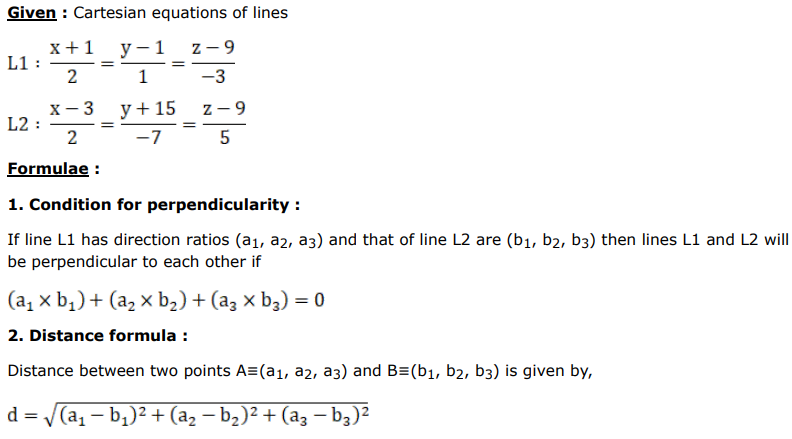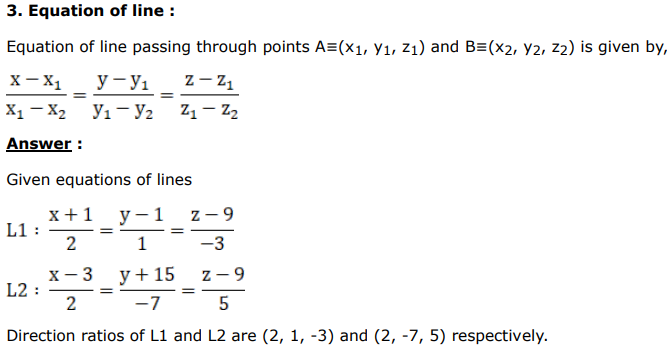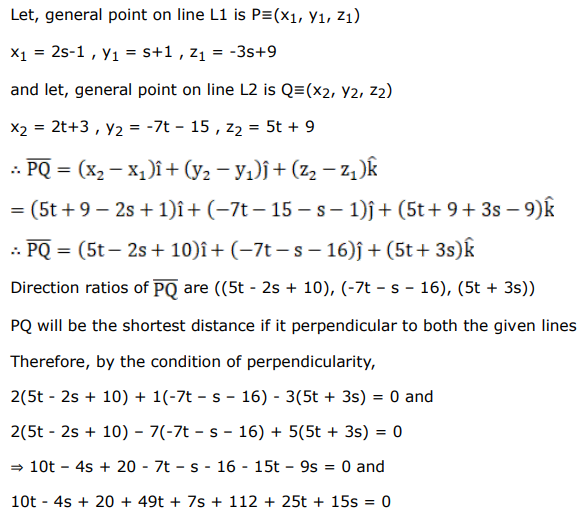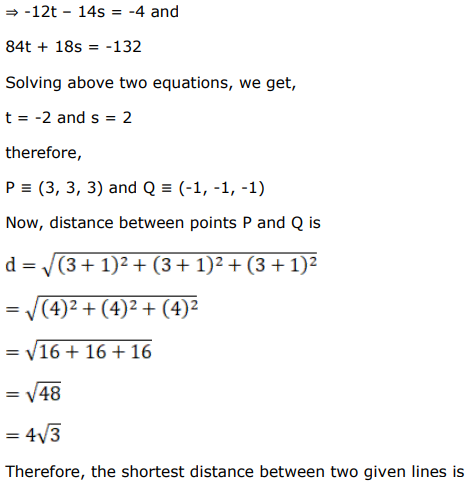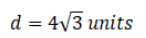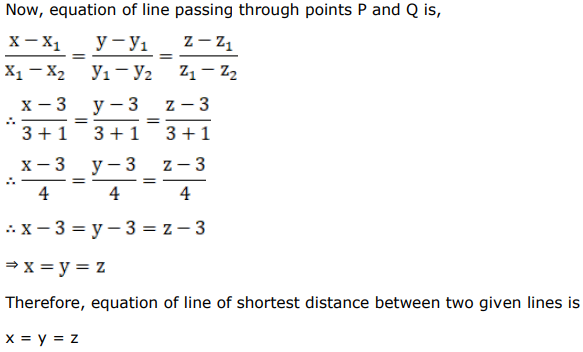# Find the length and the equations of the line of shortest distance between the lines given by:

Question:

Find the length and the equations of the line of shortest distance between the lines given by:

$\frac{x+1}{2}=\frac{y-1}{1}=\frac{z-9}{-3}$ and $\frac{x-3}{2}=\frac{y+15}{-7}=\frac{z-9}{5}$.

Solution: